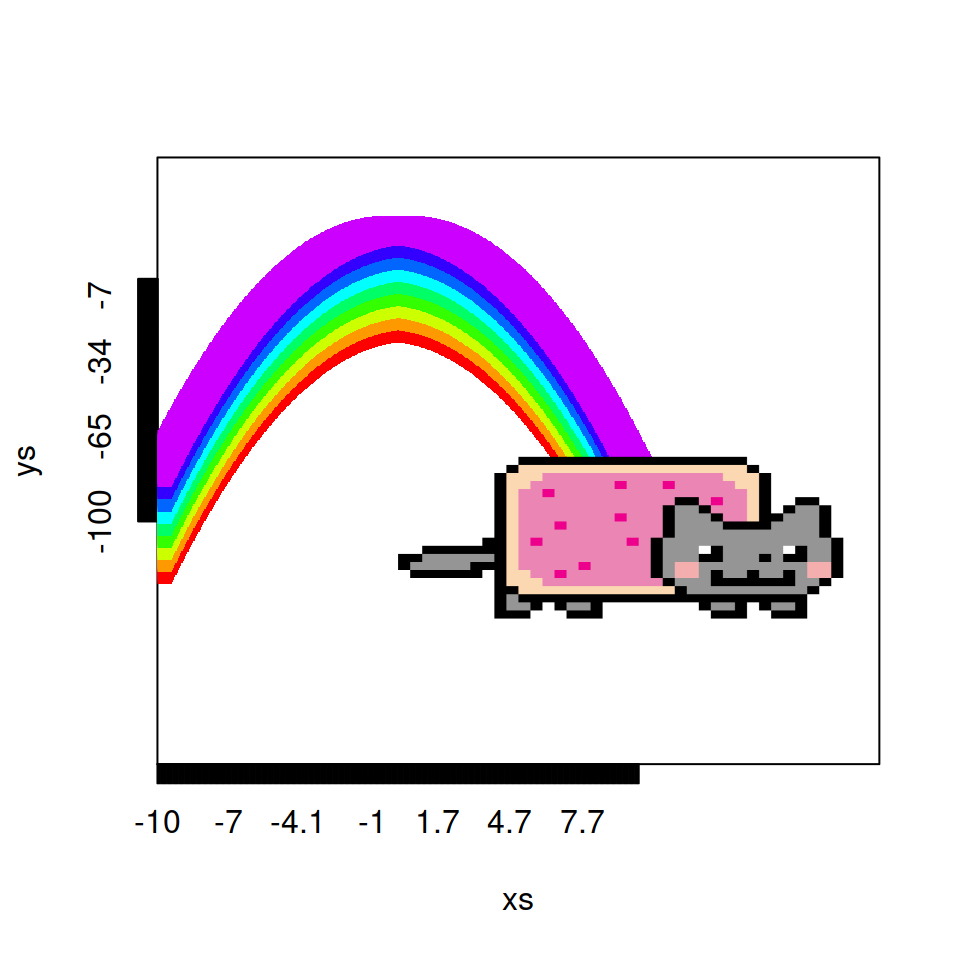# Adding cats to R plots with CatterPlots

David Gibbs

## `catplot` function

The `CatterPlots` package contains several functions to create plots with cats. The most basic function is `catplot`, which can be used to create a plot with a single cat image. Note that the cats can be chosen selecting a number between 1 and 11 (see the following section for clarification).

``````# install.packages("remotes")
# remotes::install_github("Gibbsdavidl/CatterPlots")
library(CatterPlots)

# Sample data
x <- seq(0, 15, 0.5)
y <- sin(x)

catplot(x, y,
cat = 2,       # Cat type
catcolor = 2,  # Color of the cat
type = "line", # "line" or "justcats"
linecolor = 1) # Color of the line``````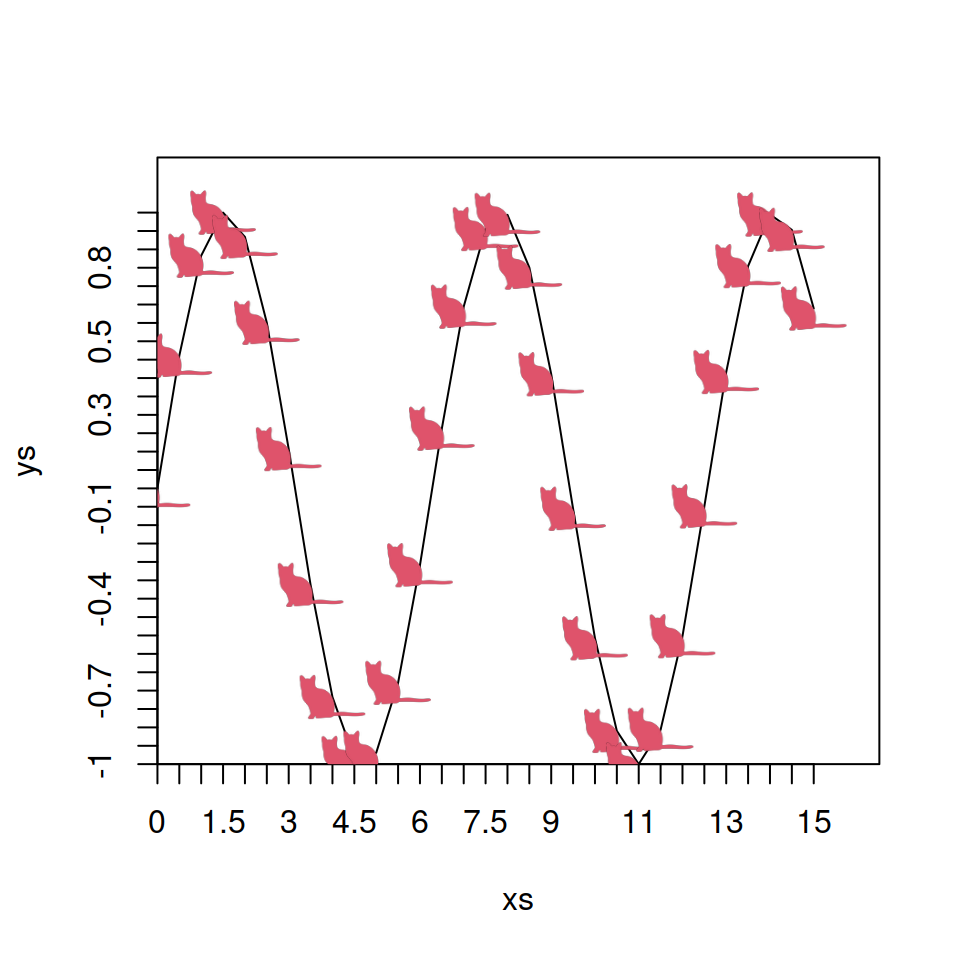In case you want to add more cats over your previous chart you can use the `cats` function, which behaves similar to the `points` function, but will need to pass the `catplot` object to the function and the new coordinates and arguments.

``````# install.packages("remotes")
# remotes::install_github("Gibbsdavidl/CatterPlots")
library(CatterPlots)

# Sample data
x <- seq(0, 15, 0.5)
y <- sin(x)

obj <- catplot(x, y,
cat = 2,
catcolor = 2,
type = "line")

cats(obj,      # catplot object
4.5, 0.8, # Coordinates
cat = 3,
catcolor = 3)``````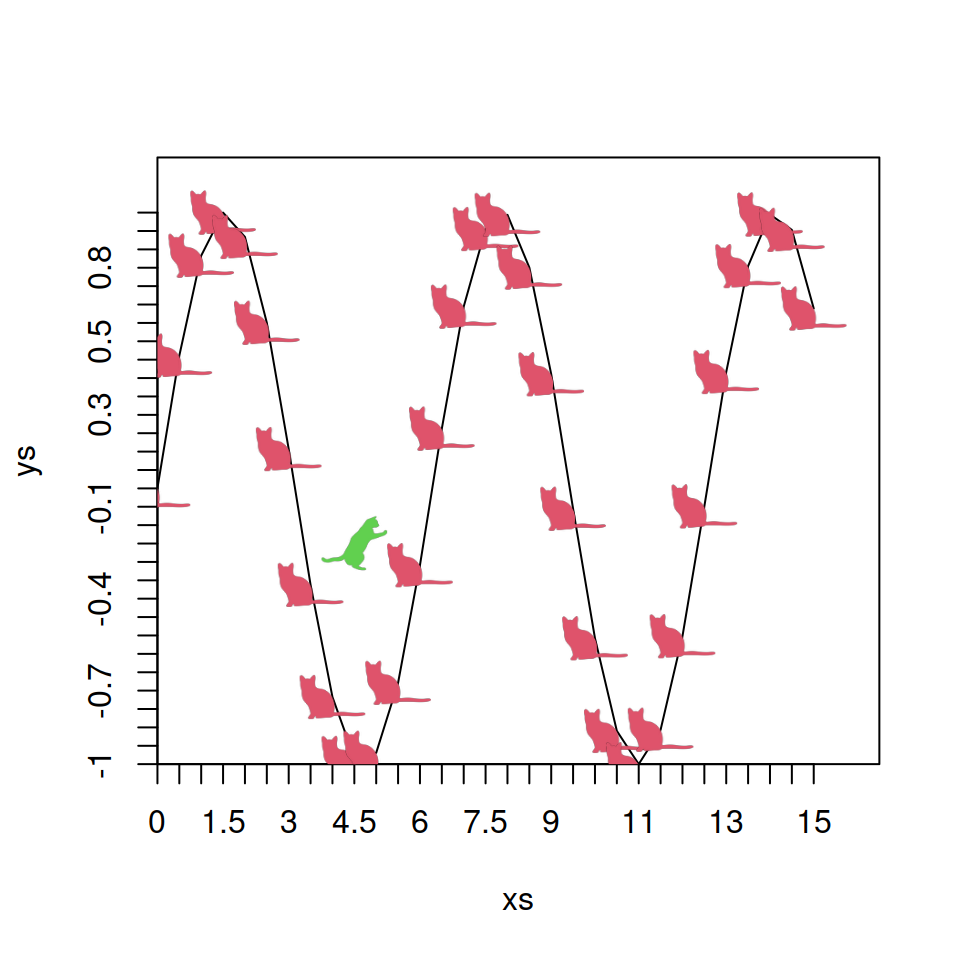Additionally, the `morecats` function allows adding even more cats with different colors and shapes.

``````# install.packages("remotes")
# remotes::install_github("Gibbsdavidl/CatterPlots")
library(CatterPlots)

# Sample data
x <- seq(0, 15, 0.5)
y <- sin(x)

obj <- catplot(x, y,
cat = 2,
catcolor = 2,
type = "line")

morecats(obj,       # catplot object
x, cos(x), # Coordinates
cat = 1:11,
catcolor = 1:11,
type = "line")``````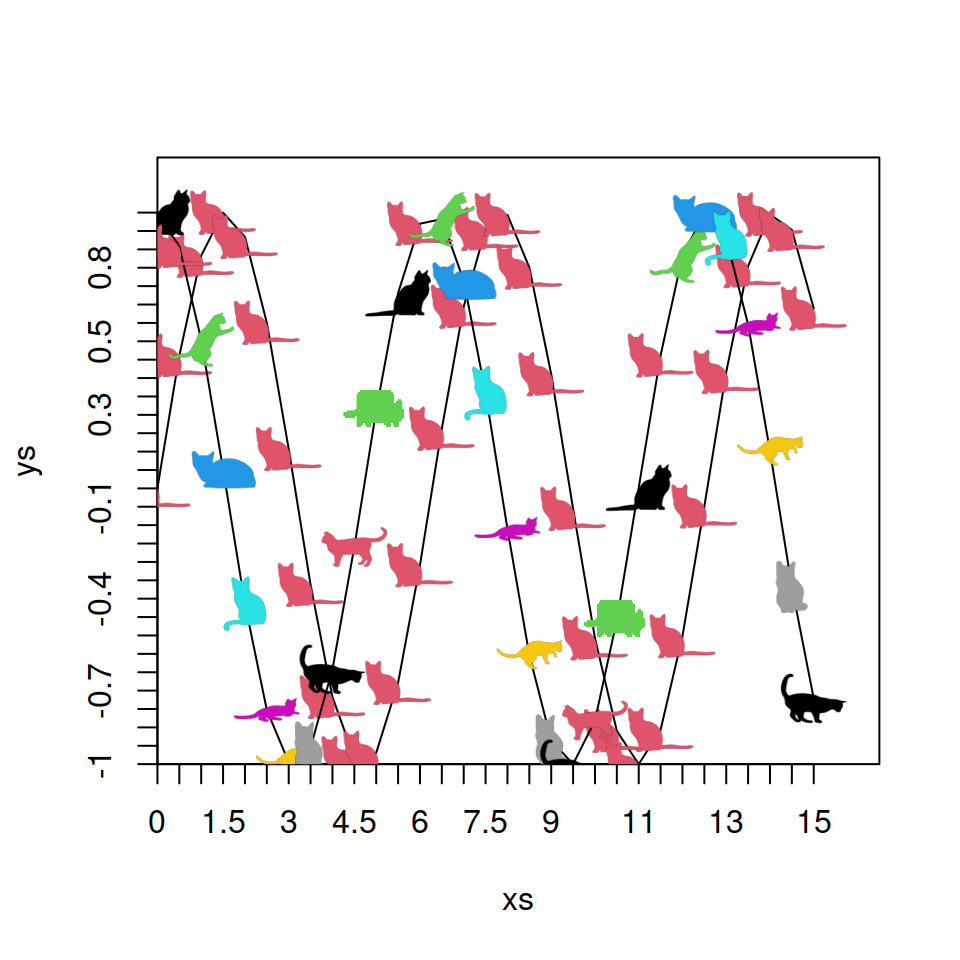## `multicat` function

An alternative to `catplot` is `multicat`. This function allows specifying more than one cat type at once. As it is possible to create the same output as with `catplot`, we would recommend using this function, as it provides more flexibility.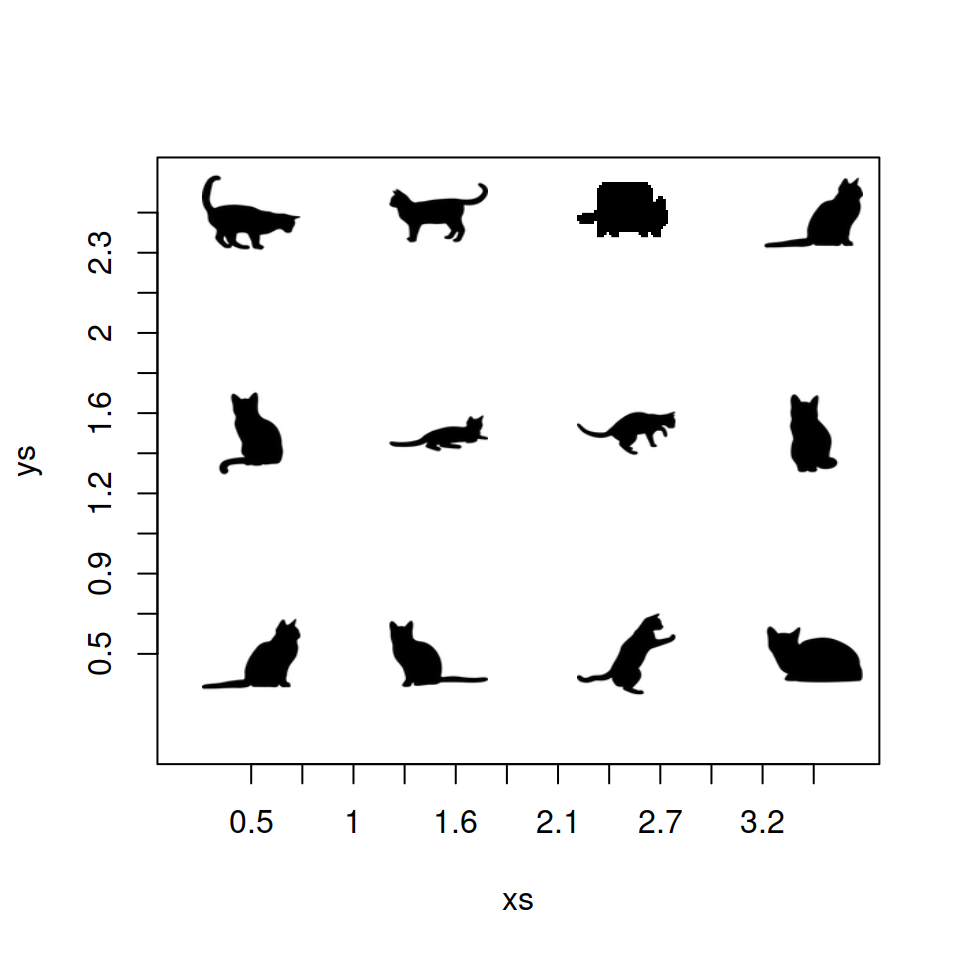``````# install.packages("remotes")
# remotes::install_github("Gibbsdavidl/CatterPlots")
library(CatterPlots)

grid <- expand.grid(0.5:3.5, 0.5:2.5)
x <- grid\$Var1
y <-  grid\$Var2

multicat(xs = x, ys = y, # Coordinates
cat = 1:11,     # Cat type
catcolor = 1,   # Cat color
size = 0.15)    # Cat size``````

## `rainbowCats` function

Finally, the package contains a function named `rainbowCats` to create a Nyan Cat plot in R, as shown below:

``````# install.packages("remotes")
# remotes::install_github("Gibbsdavidl/CatterPlots")
library(CatterPlots)

# Data
x <- -10:10
y <- -x ^ 2

# Plot
rainbowCats(x, y,